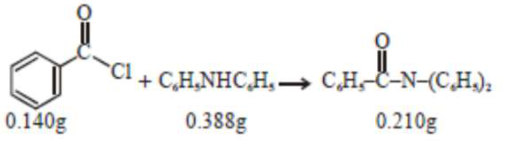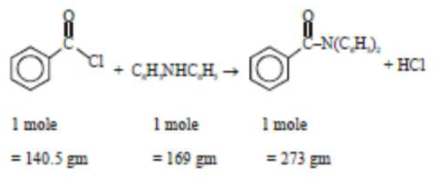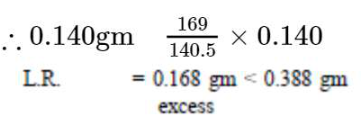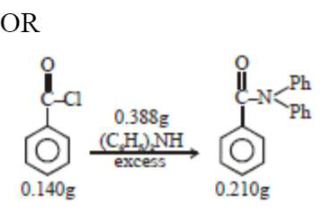# Consider the above reaction.Question:Consider the above reaction. The percentage yield of amide product is __________________ (Round off to the Nearest Integer).

(Given : Atomic mass: $\mathrm{C}: 12.0 \mathrm{u}, \mathrm{H}: 1.0 \mathrm{u}$ $\mathrm{N}: 14.0 \mathrm{u}, \mathrm{O}: 16.0 \mathrm{u}, \mathrm{Cl}: 35.5 \mathrm{u})$

Solution:

(77)$\therefore$ Theoretical amount of given product formed

$=\frac{273}{140.5} \times 0.140=0.272 \mathrm{gm}$

But its actual amount formed is $0.210 \mathrm{gm}$. Hence, the percentage yield of product.

$=\frac{0.210}{0.272} \times 100=77.20 \approx 77$Mole of $\mathrm{Ph}-\mathrm{CoCl}=\frac{0.140}{140}=10^{-3} \mathrm{~mol}$

Mole of $\mathrm{Ph}-\mathrm{C}-\mathrm{N}(\mathrm{Ph})_{2}$, that should be obtained by mol-mol analysis

$=10^{-3} \mathrm{~mol}$ Theoritical mass of product $=10^{-3} \times 273=273 \times 10^{-3} \mathrm{~g}$

Observed mass of product $=210 \times 10^{-3} \mathrm{~g}$

\%yield of product $=\frac{210 \times 10^{-3}}{273 \times 10^{-3}} \times 100=76.9 \%=77$